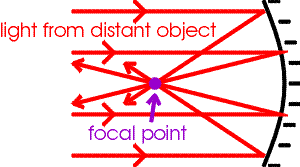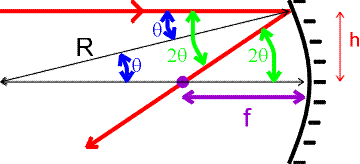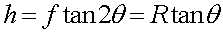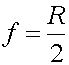Focal points of curved mirrorsMirrors can focus light. Focusing light is necessary for making images with film or recorders. Of course, lenses are more common, but mirrors are also used, e.g. the Hubble space telescope. To understand focusing, we first consider light rays from a distant object and show how the light from the object that hits the mirror can be focused to a single point, the focal point.To demonstrate that all lines regardless of h will be focused at a single point, we consider a spherical mirror with a radius of curvature R. Light that hits the surface an angle q from the normal will reflect off at an angle of 2q from the normal. Some trigonometry will now give the focal length in terms of R.For small angles, tanq approximately equals q and tan2q approximately equals 2q, thusThe fact that this crossing point is independent of h, shows that all horizontal rays will be reflected through the same point. Here, we have assumed the mirror is spherical and that the angle is small, a parabolic mirror would focus light through the same point even for large angles. Aside from telescopes, such focusing can be used by solar collectors to bring light from a large area onto a single point to be converted into electrical energy.